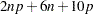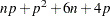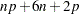Computational Resources

The various algorithms need different amounts of memory for working space. Let p be the number of parameters estimated and n be the number of observations used in the model estimation.

For the simplex algorithm, the minimum working space (in bytes) needed isfor the interior point algorithm,and for the smoothing algorithm,For the last two algorithms, if you want to use preprocessing, an extra amountis needed.

If sufficient space is available, the input data set is kept in memory; otherwise, the input data set is reread as necessary, and the execution time of the procedure increases substantially.